# Hyers-Ulam-Rassias stability

(diff) ← Older revision | Latest revision (diff) | Newer revision → (diff)

Hyers–Ulam stability

In almost-all areas of mathematical analysis one can ask the following question: "When is it true that a mathematical object satisfying a certain property approximately must be close to an object satisfying the property exactly?"

If one applies this question to the case of functional equations, one can particularly ask when the solutions of an equation differing slightly from a given one must be close to a solution of the given equation. The stability problem of functional equations originates from such a fundamental question.

In 1940, S.M. Ulam [a29] raised a question concerning the stability of homomorphisms: Let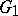be a group and let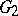be a metric group with a metric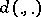. Given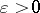, does there exist a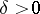such that if a function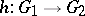satisfies the inequalityfor all, then there is a homomorphism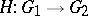with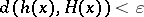for all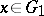?

In the following year 1941, D.H. Hyers [a11] gave a partial solution to Ulam's question. Hyers' theorem says that if a function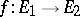defined between Banach spaces (cf. also Banach space) satisfies the inequalityfor all, then there exists a unique additive functionwith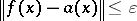for all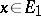.

For the above case one says that the additive Cauchy equation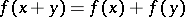has the Hyers–Ulam stability on.

Hyers explicitly constructed the additive functiondirectly from the given functionby the formula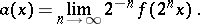This method is called a direct method and is a powerful tool for studying the stability of several functional equations. It is often used to construct a certain function which is a solution of a given functional equation.

Following its appearance, Hyers' theorem was further extended in various directions (see [a3], [a5], [a7], [a8], [a14], [a15], [a20], [a21], [a25], [a26], [a27]). In particular, Th.M. Rassias [a20] considered a generalized version of it in which the Cauchy difference is allowed to become unbounded. He assumed that a functionbetween Banach spaces satisfies the inequalityfor some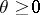,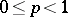and for all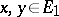. Functions such asare called approximately additive functions. Using a direct method, he proved that in this case too there exists a unique additive functionsuch thatfor all, where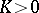depends onas well as on. This phenomenon of Hyers–Ulam–Rassias stability was later extended to alland generalizations of it were given (see [a7], [a8], [a14], [a15], [a25], [a16]). In general, Hyers–Ulam stability is a special case of Hyers–Ulam–Rassias stability.

## Superstability.

An equation involving homomorphisms is called superstable if each approximate homomorphism is actually a true homomorphism. For example, in [a2] it is proved that if a real-valued functiondefined on a vector spacesatisfies the inequality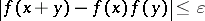for someand for all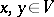, then eitheris a bounded function or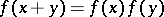for all. This result was further generalized in [a1] and [a28] (cf. also [a9], [a10]). Superstability phenomena can also be regarded as special cases of Hyers–Ulam–Rassias stability.

For results concerning the stability of other equations, see [a4], [a17], [a18], [a19], [a22], or the references listed in [a12], [a30]. The survey papers [a13], [a6], [a16], [a23], [a24] contain general information on stability. See [a12], [a30] for a comprehensive introduction to the general theory of Hyers–Ulam–Rassias stability of functional equations.

In the same vein there is a theory of almost isomorphisms of Banch algebras,-perturbations of the multiplication and-isometries of Banach algebras. See [a31] for a selection of results.

How to Cite This Entry:
Hyers-Ulam-Rassias stability. Encyclopedia of Mathematics. URL: http://encyclopediaofmath.org/index.php?title=Hyers-Ulam-Rassias_stability&oldid=11833
This article was adapted from an original article by Soon-Mo Jung (originator), which appeared in Encyclopedia of Mathematics - ISBN 1402006098. See original article